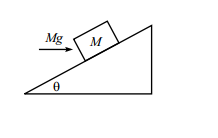# Block on Incline (2)A block of mass M rests on an fixed incline plane of angle $\theta$. A horizontal force of magnitude Mg is applied on the block, If the coefficient of static friction of the incline be $0.5$ . For what ranges of $\theta$ will the block still remain at rest?

Note : The answer is of the form $a\leq tan\theta\leq b$ Find $ab$

×